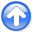Applications of plane-wave destruction filtersNext: Application examples Up: Fomel: Plane-wave destructors Previous: High-order plane-wave destructors

# Slope estimation

Let us denote bythe operator of convolving the data with the 2-D filterof equation (12), assuming the local slopeis known. In order to determine the slope, we can define the least-squares goal(13)

whereis the known data and the approximate equality implies that the solution is found by minimizing the power of the left-hand side. Equations (9) and (10) show that the slopeenters in the filter coefficients in an essentially non-linear way. However, one can still apply the linear iterative optimization methods by an analytical linearization of equation (13). The linearization (also known as the Gauss-Newton iteration) implies solving the linear system(14)

for the slope increment. Hereis the initial slope estimate, andis a convolution with the filter, obtained by differentiating the filter coefficients ofwith respect to. After system (14) is solved, the initial slopeis updated by addingto it, and one can solve the linear problem again. Depending on the starting solution, the method may require several non-linear iterations to achieve an acceptable convergence.

The slopein equation (14) does not have to be constant. We can consider it as varying in both time and space coordinates. This eliminates the need for local windows but may lead to undesirably rough (oscillatory) local slope estimates. Moreover, the solution will be undefined in regions of unknown or constant data, because for these regions the local slope is not constrained. Both these problems are solved by adding a regularization (styling) goal to system (14). The additional goal takes the form(15)

whereis an appropriate roughening operator andis a scaling coefficient. For simplicity, I choseto be the gradient operator. More efficient and sophisticated helical preconditioning techniques are available (Fomel, 2001; Claerbout, 1998; Fomel and Claerbout, 2002).

In theory, estimating two different slopesandfrom the available data is only marginally more complicated than estimating a single slope. The convolution operator becomes a cascade ofand, and the linearization yields(16)

The regularization condition should now be applied to bothand:(17)(18)

The solution will obviously depend on the initial values ofand, which should not be equal to each other. System (16) is generally underdetermined, because it contains twice as many estimated parameters as equations: The number of equations corresponds to the grid size of the data, while characterizing variable slopesandon the same grid involves two gridded functions. However, an appropriate choice of the starting solution and the additional regularization (17-18) allow us to arrive at a practical solution.

The application examples of the next section demonstrate that when the system of equations (14-15) or (16-18) are optimized in the least-squares sense in a cycle of several linearization iterations, it leads to smooth and reliable slope estimates. The regularization conditions (15) and (17-18) assure a smooth extrapolation of the slope to the regions of unknown or constant data.Applications of plane-wave destruction filtersNext: Application examples Up: Fomel: Plane-wave destructors Previous: High-order plane-wave destructors

2014-03-29